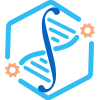### Mathematical Biosciences and Engineering

2015, Issue 5: 983-1006. doi: 10.3934/mbe.2015.12.983

# Multi-host transmission dynamics of schistosomiasis and its optimal control

• Received: 01 December 2014 Accepted: 29 June 2018 Published: 01 June 2015
• MSC : Primary: 92B05, 92D30.

• -->
In this paper we formulate a dynamical model to study the transmission dynamics of schistosomiasis in humans and snails. We also incorporate bovines in the model to study their impact on transmission and controlling the spread of Schistosoma japonicum in humans in China. The dynamics of the model is rigorously analyzed by using the theory of dynamical systems. The theoretical results show that the disease free equilibrium is globally asymptotically stable if $\mathcal R_0<1 and="" if="" mathcal="" r_0="">1$ the system has only one positive equilibrium. The local stability of the unique positive equilibrium is investigated and sufficient conditions are also provided for the global stability of the positive equilibrium. The optimal control theory are further applied to the model to study the corresponding optimal control problem. Both analytical and numerical results suggest that: (a) the infected bovines play an important role in the spread of schistosomiasis among humans, and killing the infected bovines will be useful to prevent transmission of schistosomiasis among humans; (b) optimal control strategy performs better than the constant controls in reducing the prevalence of the infected human and the cost for implementing optimal control is much less than that for constant controls; and (c) improving the treatment rate of infected humans, the killing rate of the infected bovines and the fishing rate of snails in the early stage of spread of schistosomiasis are very helpful to contain the prevalence of infected human case as well as minimize the total cost.

Citation: Chunxiao Ding, Zhipeng Qiu, Huaiping Zhu. Multi-host transmission dynamics of schistosomiasis and its optimal control[J]. Mathematical Biosciences and Engineering, 2015, 12(5): 983-1006. doi: 10.3934/mbe.2015.12.983

### Related Papers:

• In this paper we formulate a dynamical model to study the transmission dynamics of schistosomiasis in humans and snails. We also incorporate bovines in the model to study their impact on transmission and controlling the spread of Schistosoma japonicum in humans in China. The dynamics of the model is rigorously analyzed by using the theory of dynamical systems. The theoretical results show that the disease free equilibrium is globally asymptotically stable if $\mathcal R_0<1 and="" if="" mathcal="" r_0="">1$ the system has only one positive equilibrium. The local stability of the unique positive equilibrium is investigated and sufficient conditions are also provided for the global stability of the positive equilibrium. The optimal control theory are further applied to the model to study the corresponding optimal control problem. Both analytical and numerical results suggest that: (a) the infected bovines play an important role in the spread of schistosomiasis among humans, and killing the infected bovines will be useful to prevent transmission of schistosomiasis among humans; (b) optimal control strategy performs better than the constant controls in reducing the prevalence of the infected human and the cost for implementing optimal control is much less than that for constant controls; and (c) improving the treatment rate of infected humans, the killing rate of the infected bovines and the fishing rate of snails in the early stage of spread of schistosomiasis are very helpful to contain the prevalence of infected human case as well as minimize the total cost.###### 通讯作者: 陈斌, bchen63@163.com
• 1.

沈阳化工大学材料科学与工程学院 沈阳 1101422.194 3.0

Article outline

## Other Articles By Authors

• On This SiteDownLoad:  Full-Size Img  PowerPoint